Previous Year Questions: Circles - 2

# Previous Year Questions: Circles - 2 Notes | Study Mathematics (Maths) Class 10 - Class 10

## Document Description: Previous Year Questions: Circles - 2 for Class 10 2022 is part of Mathematics (Maths) Class 10 preparation. The notes and questions for Previous Year Questions: Circles - 2 have been prepared according to the Class 10 exam syllabus. Information about Previous Year Questions: Circles - 2 covers topics like and Previous Year Questions: Circles - 2 Example, for Class 10 2022 Exam. Find important definitions, questions, notes, meanings, examples, exercises and tests below for Previous Year Questions: Circles - 2.

Introduction of Previous Year Questions: Circles - 2 in English is available as part of our Mathematics (Maths) Class 10 for Class 10 & Previous Year Questions: Circles - 2 in Hindi for Mathematics (Maths) Class 10 course. Download more important topics related with notes, lectures and mock test series for Class 10 Exam by signing up for free. Class 10: Previous Year Questions: Circles - 2 Notes | Study Mathematics (Maths) Class 10 - Class 10
 1 Crore+ students have signed up on EduRev. Have you?

Q.15. The difference between the radii of the smaller circle and the larger circle is 7 cm and the difference between the areas of the two circles is 1078 sq. cm. Find the radius of the smaller circle.    [CBSE Delhi 2017 (C)]
Ans. Given: r2 - r1 = 7 (r2 > r1) ...(i)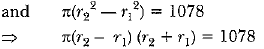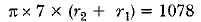(From equation (i))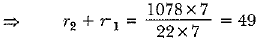..... (ii)
Adding (i) and (ii), we get
2r2 = 56
⇒ r2 = 28 cm
Also, r1 = 21 cm (From equation (i))
∴ Radius of simaller circle = 21 cm.

Q.16. If from an external point P of a circle with centre 0, two tangents PQ and PR are drawn such that QPR = 120°, prove that 2PQ = PO.      [CBSE Delhi 2014, (F) 2016]
Ans. Given, ∠QPR = 120°
Radius is perpendicular to the tangent at the point of contact.
∠OQP = 90° ⇒ ∠QPO = 60°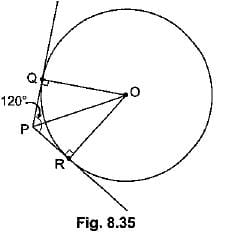(Tangents drawn to a circle from an external point are equally inclined to the segment, joining the centre to that point.)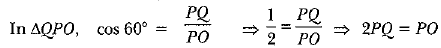Q.17. In Fig. 8.42, AP and BP are tangents to a circle with centre O, such that AP = 5 cm and ∠APS = 60°. Find the length of chord AB.     [CBSE Delhi 2016]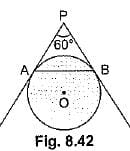Ans. PA = PB (Tangents from an external point are equal)
and ∠APB = 60°
⇒ ∠PAB = ∠PBA = 60°
∴ ΔPAB is an equilateral triangle.
Hence AB = PA = 5 cm.

Q.18. In Fig. 8.43 from an external point P, two tangents PT and PS are drawn to a circle with centre O and radius r. If OP = 2r, show that ∠OTS = ∠OST = 30°.      [CBSE (AI) 2016]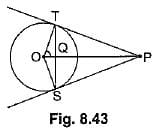Ans. Let ∠TOP = θ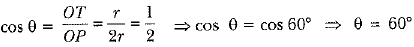Hence, ∠TOS = 120°
In ∠OTS, OT = OS    (Radii of circle)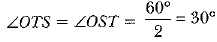Q.19. In Fig. 8.44, are two concentric circles of radii 6 cm and 4 cm with centre O. If AP is a tangent to the larger circle and BP to the smaller circle and length of AP is 8 cm, find the length of BP.     [CBSE (F) 2016]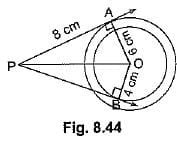Ans. OA = 6 cm, OB = 4 cm, AP = 8 cm
OP2 = OA2 + AP2 = 36 + 64 = 100
⇒ OP = 10 cm
BP2 = OP2 - OB2 = 100 - 16 = 84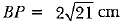Q.20. From an external point P, tangents PA and PR are drawn to a circle with centre O. If ∠PAB = 50°, then find ∠AOB.      [CBSE Delhi 2016]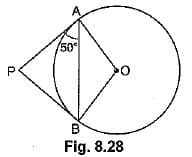Ans. ∵ PA = PB ⇒ ∠BAP = ∠ABP = 50°
∴  ∠APB = 180° - 50° - 50° = 80°
and ∠AOB = 180° - 80° = 100°

Q.21. In Fig. 8.29, PQ is a tangent at a point C to a circle with centre O. If AB is a diameter and ∠CAB = 30°, find ∠PCA.      [CBSE (AI) 2016]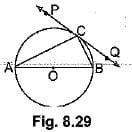Ans. ∠ACB = 90° (Angle in the semicircle)
∠CAB = 30° (given)
In ΔABC,
90° + 30° + ∠ABC = 180°
⇒ ΔABC = 60°
Now, ∠PCA = ∠ABC (Angles in the alternate segment)
∴  ∠PCA = 60°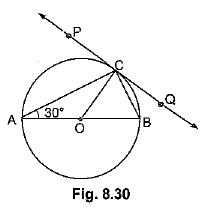OR
Construction: Jo in 0 to C.
∠PCO = 90° (∵ Line joining centre to point of contact is perpendicular to PQ)
In ΔAOC, OA = OC (Radii of circle)
∴ ∠OAC = ∠OCA = 30° (Equal sides have equal opp. angles)
Now, ∠PCA = ∠PCO - ∠ACO
= 90° - 30° = 60°

Q.22. Two tangents PA and PB are drawn to the circle with centre O, such that ∠APB = 120°. Prove that OP = 2AP.       (Foreign 2016)
Ans.  Given. A circle C(0, r). PA and PB are tangents to the circle from point P, outside the circle such that ∠APB = 120°. OP is joined.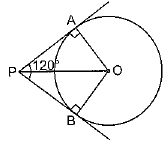To Prove. OP = 2AP.
Construction. Join OA and OB.
Proof. Consider Δs PAO and PBO
PA = PB [Tangents to a circle, from a point outside it, are equal.]
OP = OP [Common]
∠OAP = ∠OBP = 90°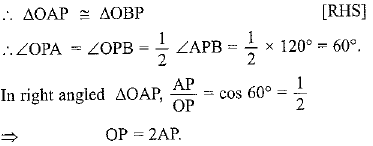Long Answer Type Questions

Q.1. Prove that the parallelogram circumscribing a circle is a rhombus.      [CBSE Delhi 2014; CBSE 2019 (30/5/1)]
Ans. Let ABCD be a parallelogram such that its sides touch a circle with centre O.
We know that the tangents to a circle from an exterior point are equal in length.
Therefore, we have
AP = AS             (Tangents from A)      ... (i)
BP = BQ             (Tangents from B)     ... (ii)
CR = CQ              (Tangents from C)     ... (iii)
And DR = DS      (Tangents from D) ... (iv)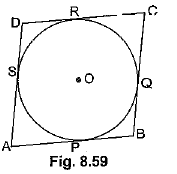Adding (i), (ii), (iii) and (iv), we have
(AP + BP) + (CR + DR) = (AS + DS) + (BQ + CQ)
AB + CD = AD + BC
AB + AB = BC + BC      (∵ ABCD is a parallelogram ∴ AB = CD, BC = DA)
2AB = 2BC ⇒ AB = BC
Thus, AB = BC = CD = AD
Hence, ABCD is a rhombus.

Q.2. Prove that the tangent drawn at any point of a circle is perpendicular to the radius through the point of contact.    [AI (C) 2017]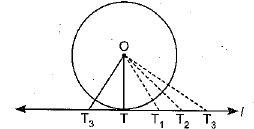Ans. Given: A line T tangent to the circle at point T and O is the centre of circle.
To prove: OT ⊥ l
Construction: Take point T1,T2 and T3 on line l and join OT1, OT2, OT3
Proof: We observe that points T1, T2, T3 lie outside the circle, whereas point T lies on the circle.
Hence OT1 > OT
OT2 > OT
OT3 > OT
All distances OT1, OT2, OT3 are greater than OT.
Only OT is the shortest distance.
Also OT = r
Hence r is the shortest distance from tangent l of the circle to the centre as we know that shortest distance between the point on line is perpendicular distance
So, OT ⊥ l

Q.3. In Fig., PQ is a chord of length 8 cm of a circle of radius 5 cm. The tangents drawn at P and Q intersect at T. Find the length of TP.    [AI (C) 2017, Foreign 2015]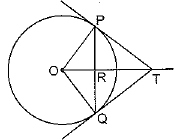Ans. Given: PQ is a chord of length 8 cm
Radius OP = 5 cm. PT and QT are tangents to the circle.
To find: TP
OT is perpendicular bisector of PQ
∠ORP = 90°
(Line joining the centre of circle to the common point of two tangents drawn to circle is perpendicular bisector of line joining the point of contact of the tangents.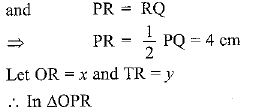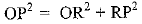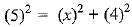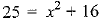x2 = 16
⇒ x = 3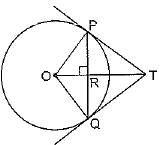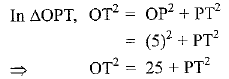(OR + RT)2 = 25 + PT2
(3 + y)2 = 25 + PT2
9 + y2 + 6y = 25 + PT2         .......(i)
In ΔPRT, TP2 = PR2 + RT2
⇒ PT2 = (4)2 + (y)2       .......(ii)
Put value of PT2 in eq (i)
9 + y2 + 6y = 25 + 16 + y2
6y = 25 + 16 - 9
6y = 32
y = 32/6 = 16/3 cm
Putting y = 16/3 cm in eq (ii), we get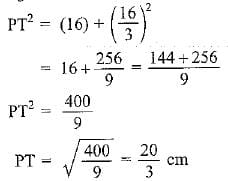Q.4. Prove that the lengths of two tangents drawn from an external point to a circle are equal. [CBSE, Delhi 2014, (F) 2014, Delhi 2016, (AI) 2016, (F) 2016, CBSE Delhi 2017, (AI) 2017, (F) 2017, Delhi 2017 (C)]
Ans. Given: AP and AQ are two tangents from a pointed to a circle C (O, r).
To Prove: AP = AQ
Construction: Join OP, OQ and OA.
Proof: In order to prove that AP = AQ, we shall first prove that ΔOPA ≅ ΔOQA.
Since a tangent at any point of a circle is perpendicular to the radius through the point of contact.
∴ OP ⊥ AP and OQ ⊥ AQ
⇒ ∠OPA = ∠OQA = 90°    ........(i)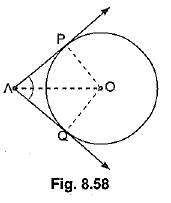Now, in right triangles OPA and OQA, we have
OP = OQ    (Radii of a circle)
∠OPA = ∠OQA    (Each 90°)
and OA = OA    (Common)
So, by RHS-criterion of congruence, we get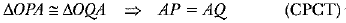Hence, lengths of two tangents from an external point are equal.

Q.5. In Fig. 8.62, two equal circles, with centres O and O', touch each other at X.OO' produced meets the circle with centre O' at A. AC is tangent to the circle with centre O, at the point C. O'D is perpendicular to AC. Find the value of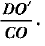[CBSE (AI) 2016]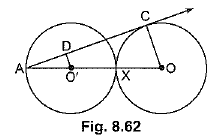Ans. AC is tangent to circle with centre O.
Thus ∠ACO = 90°
In ΔAO'D and ΔAOC
∠ADO' = ∠ACO = 90º
∠A = ∠A  (Common)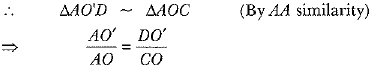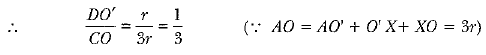Q.6. In Fig. 8.63, O is the centre of a circle of radius 5 cm. T is a point such that OT = 13 cm and OT intersects circle at E. If AB is a tangent to the circle at E, find the length of AB, where TP and TQ are two tangents to the circle.     [CBSE Delhi 2016]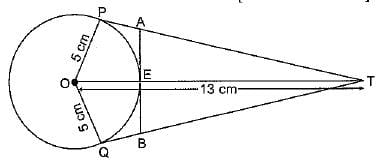Ans.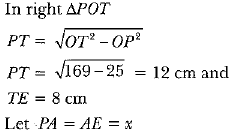(Tangents from an external point to a circle are equal)
In right ΔAET.
TA2 = TE2 + EA2
(12 - x)2 = 64 + x2        144 + x2 - 24x = 64 + x2
x = 80/24    ⇒ x = 3.3 cm
Thus, AB = 6.6 cm

The document Previous Year Questions: Circles - 2 Notes | Study Mathematics (Maths) Class 10 - Class 10 is a part of the Class 10 Course Mathematics (Maths) Class 10.
All you need of Class 10 at this link: Class 10

## Mathematics (Maths) Class 10

53 videos|369 docs|138 tests
 Use Code STAYHOME200 and get INR 200 additional OFF

## Mathematics (Maths) Class 10

53 videos|369 docs|138 tests

Track your progress, build streaks, highlight & save important lessons and more!

,

,

,

,

,

,

,

,

,

,

,

,

,

,

,

,

,

,

,

,

,

;